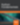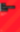# Ruby Interview Questions: Problem Walkthroughs

Ruby

Share:

#### Free JavaScript Book!Write powerful, clean and maintainable JavaScript.

RRP \$11.95We’ve talked a bit about interviewing with Ruby and the basic tools of the game. In this article, we will consider some harder interview questions that ask us to apply some of the concepts we have learned thus far. The point here isn’t just to learn how to implement the solutions to the problems, but the train of thought that leads to a solution.

The problem you are presented with while you stand nervously at the whiteboard (by the way, whiteboard coding sucks) might be very different from the stuff you’ve already seen, but if you truly understand the motivation behind the solutions, you’ll be fine. Without further ado, let’s look at our first problem.

## Closest Ancestors

Here’s the first problem:

Given two nodes within a binary tree, find their lowest common ancestor.

Where the lowest common ancestor is the first node (i.e. one with minimum distance) that both given nodes have in common as an ancestor. OK, let’s think through this thing.

Say we’re dealing with nodes N1 and N2. If want to find the lowest common ancestor, we can imagine moving up the list of ancestors from both N1 and N2 and checking if either of them match. However, what order to go up the ancestors in (e.g. go through all of N1’s first or N2’s first or do it intermittently) is an issue, especially if N1 is at the very “left” side of the tree and N2 is at the far right. Let’s try thinking about this another way.

Say we’ve found the solution, i.e. the lowest common ancestor is the node R. Then, what properties does does R have to satisfy? It must have N1 as a descendant and it must also have N2 as a descendant. If only one or neither of these is true, then we know that the node cannot be the least common ancestor. Furthermore, R must be the lowest node within the tree that has this property. Looking at the solving condition gives us an idea: what if we traverse the tree, check if each node has N1 and N2 as descendants as part of our traversal and return (i.e. bubble up through our recursion) the lowest node that satisfies this property. There’s just one issue: what if N2 is one of the children (or descendants) of N1? To solve this problem and provide a base case for the recursion, we check if the current node is either N1 or N2 and, if it is, it must be the lowest common ancestor!

That explanation might seem a bit confusing and “hand wavy” but the code makes it much more clear:

``````def get_lca(root, n1, n2)
return nil if root == nil
return root if root == n1 || root == n2
left_lca = get_lca(root.left, n1, n2)
right_lca = get_lca(root.right, n1, n2)
return root if (left_lca != nil and right_lca != nil)
if left_lca != nil then return left_lca else return right_lca end
end
``````

We can test this with a simple tree of height three:

``````grandchild1 = TreeNode.new(4, nil, nil)
grandchild2 = TreeNode.new(5, nil, nil)
grandchild3 = TreeNode.new(6, nil, nil)
grandchild4 = TreeNode.new(7, nil, nil)
child1 = TreeNode.new(2, grandchild1, grandchild2)
child2 = TreeNode.new(3, grandchild3, grandchild4)
root = TreeNode.new(1, child1, child2)
puts get_lca(root, grandchild1, grandchild2).value
=> 2
puts get_lca(root, grandchild3, grandchild4).value
=> 3
puts get_lca(root, grandchild1, grandchild3).value
=> 1
``````

Let’s break down the algorithm. If the root is nil, then that means we’ve just been handed an empty tree through the recursion or by the initial call. If the root is `nil`, there can’t possibly be a lowest common ancestor for N1 and N2 (which we assume are non-nil because if they are nil, the problem does not have a well-defined answer).

Then, we have our “second” base case: we return the root if the root is either one of N1 or N2. This is due to the fact that this situation implies that the N1 or N2 must be an ancestor of the other one (i.e. N2 or N1). If we still aren’t done, then we search the left and right subtrees. If there is a non-nil return value, this means that N1 or N2 (or an ancestor of both) was found in the subtree. If the left subtree contains N1 and the right subtree contains N2, then the current root node must be the LCA (try to convince yourself of this using an inductive argument). If only one of them isn’t nil, then that side must be the correct LCA.

It’s also instructive to see the inductive proof for why this approach works. We’ve hand-waved some bits of it so an outline of it will help clear up the concept behind the algorithm. Our goal is to show that `get_lca` does in fact return the lowest common ancestor of the nodes N1 and N2. We’ll also assume that N1 is not the same as N2; this is an easy issue to resolve but will just make the argument a little bit more complicated so we’ll avoid it. Consider the base case of a tree of height two, including the root node. It is easy to verify that regardless of where N1 and N2 are placed within this tree (as children or with one of them being the root), `get_lca` will return the correct lowest common ancestor. Furthermore, `get_lca` has another property. If this tree only contains only one of N1 and N2, then `get_lca` will return the one that the tree contains.

So, let’s assume that both of these properties hold for trees of height `h`. Then, consider a tree of height `h+1` with root node `root`. We have three cases. If the root node is one of N1 and N2, then we’ve found our answer. If N1 and N2 are on two different subtrees, we handle that that calling `get_lca` on the subtrees of height `h` which should return N1 and N2 (i.e. not `nil`) and we return the root, as desired. Finally, if N1 and N2 are in the same subtree, then only one of the `get_lca`s will return non-nil and that must be the correct lowest common ancestor since we have assumed this property for the subtrees of height `h`. That proves `get_lca` is correct!

The point of having an idea of the inductive proof is not so that you can regurgitate it when someone mentions the word “induction”. But, it should help solidify your understanding of how and why the algorithm works. Take the time to understand it to the point where the proof itself comes naturally. The key property we are utilizing is that `get_lca` actually returns the correct LCA if both N1 and N2 are present in the tree it is given but it also returns which of N1 and N2 is present if only one of them is.

## Merge Sorted Lists

On to something a bit different:

Merge two sorted linked lists of integers.

Often, it’s a good idea to work with an example at first and then come with a general-case strategy. Let’s consider `[4, 5, 9, 11]` and `[6, 7, 8, 12]`. The goal is to design a method that takes these two linked lists (i.e. their head nodes) and returns the head node of a merged, sorted list. Let’s call the heads of the lists `head1` and `head2`.`4` with be the first element of our newly formed list since `4 < 6`. So, we have to keep track of the head of the merged list – let’s call it `res`. Make `res` equal to `head1` so that it contains the value of `4`. Now what?

We still have a very similar looking problem: trying to merge the lists `[5, 9, 11]` and `[7, 8, 12]`. Since it’s the same problem with different inputs, we can use recursion! Just pass along `head1.next` and `head2` along recursively! This should return a new list head which we will tack onto the end of `res`.

The algorithm that comes out of this example is actually pretty simple. Say we are given `head1` and `head2`. If either of these are `nil`, we just return the other one since we have no merging to do if one of them is empty. If not, compare the values of the two heads. Call the smaller one `small_head` and the bigger one `big_head`. Then, recursively merge `small_head.next` and `big_head` and tack this onto the end of the resulting list. Let’s this play out in code:

``````def merge_sorted(head1, head2)
end

return res
end
``````

The code pretty much reflects the description (even the variable names!) so it’s pretty easy to understand. You should try to construct an inductive argument as to why this works. Notice that the inductive argument is simpler than the one we constructed for `get_lca` since there’s only one property of `merge_sorted` we care about: the fact that it correctly merges sorted linked lists. We can test the code:

``````stack1 = Stack.new
stack1.push 11
stack1.push 9
stack1.push 5
stack1.push 4

stack2 = Stack.new
stack2.push 12
stack2.push 8
stack2.push 7
stack2.push 6

=> [4, 5, 9, 11]
=> [4, 5, 6, 7, 8, 9, 11, 12]
``````

Notice that we’re using the `Stack` class from the last article just to create the list. You’re welcome to construct it with a bunch of `Node` objects if you would like. Here’s an interesting question: what happens to the original lists? Let’s check:

``````puts merge_sorted(stack1.head, stack2.head)
=> [4, 5, 6, 7, 8, 9, 11, 12]
``````

If you run this, you’ll see that the lists are not preserved. This is due to the fact that we are merging the lists in-place. You should discuss with your interviewer the advantages and drawbacks of in-place modification and proceed accordingly. To preserve the original list, all we would have to do is create a new `res` node rather than just have it equal `small_node`.

## Binary Search Trees

Let’s take a look at an easier but reasonably popular problem:

Insert a node into a binary search tree while maintaining the properties of a binary search tree.

As you might recall, a binary search tree is a tree that satisfies the following property: each node has a value that is greater than or equal to all of its left descendants and smaller than or equal to all of its right descendants.

Since we can see “binary” and “tree” in the problem, we should probably think about doing this recursively. Given the node to insert (let’s call it N) and the root of the tree (call it R), check if N’s value is greater than the R’s value. If this is the case, then we know that N can be placed in the right subtree. If not, it can be placed in the left subtree. Let’s implement it:

``````def insert_in_bst(root, node)
return node if root == nil
if node.value > root.value
if root.right == nil
root.right = node
else
insert_in_bst root.right, node
end
else
if root.left == nil
root.left = node
else
insert_in_bst root.left, node
end
end
end
``````

All we’re doing is traversing down the tree and as soon as we find a spot where our node can “fit” (i.e. it satisfies the binary search tree property by not being too big or too small), insert it. Try to think about whether or not this will always produce a balanced binary search tree. If not, how could we write an algorithm that does that?

## Wrapping It Up

Hopefully this review of interview problems will help you score that next job using some Ruby. We’ll continue next time with a rather esoteric, but sometimes useful, data structure: the Bloom filter.### Dhaivat Pandya

I'm a developer, math enthusiast and student.

#### New books out now!Learn valuable skills with a practical introduction to Python programming!Give yourself more options and write higher quality CSS with CSS Optimization Basics.